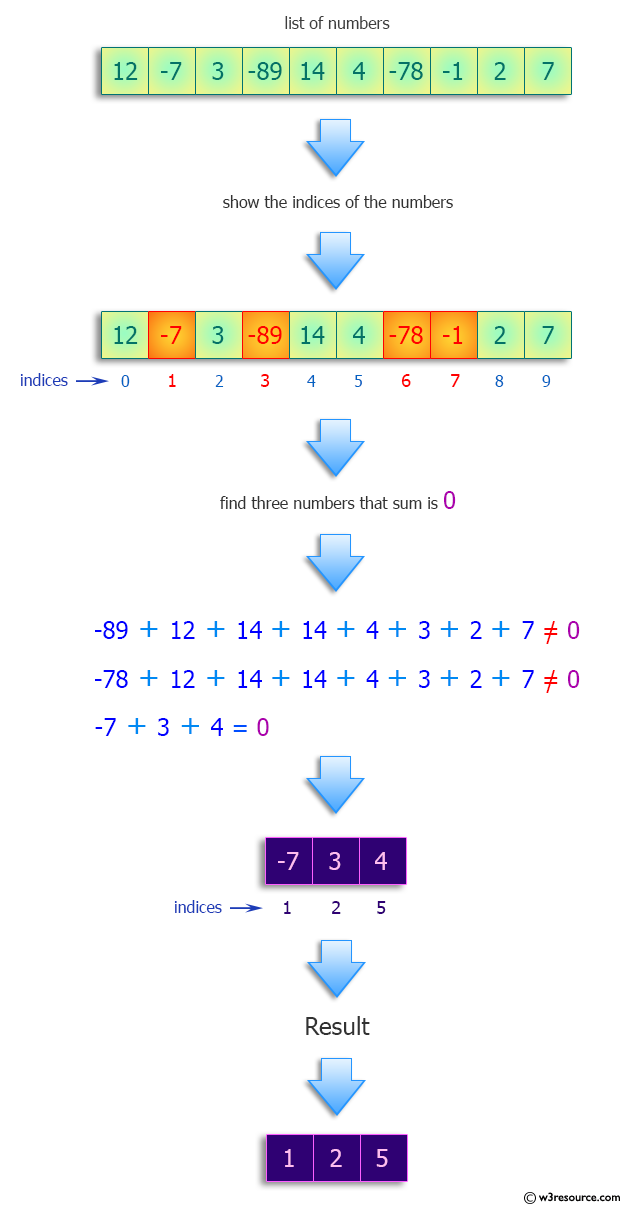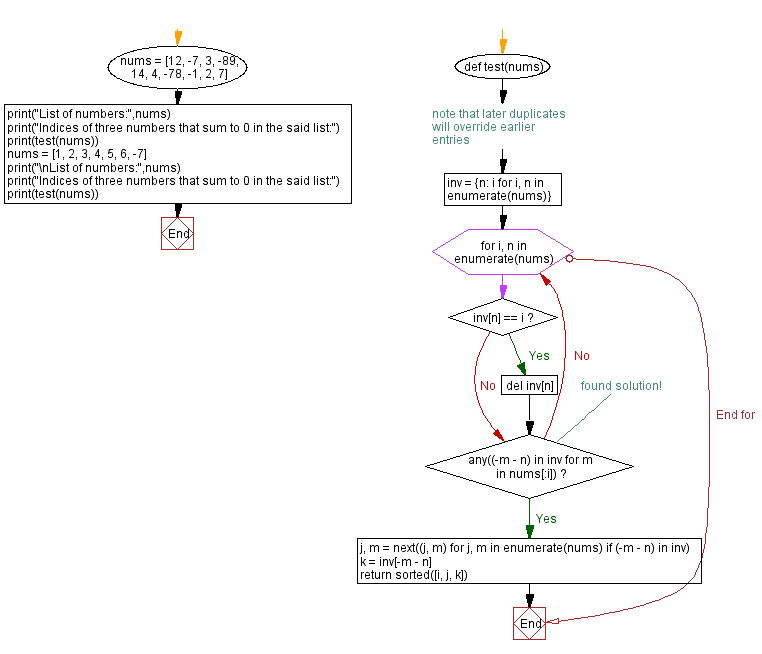﻿ Python: Find the indices of three numbers that sum to 0 in a list - w3resource# Python: Find the indices of three numbers that sum to 0 in a list

## Python Programming Puzzles: Exercise-72 with Solution

Write a Python program to find the indices of three numbers that sum to 0 in a given list of numbers.

```Input: [12, -7, 3, -89, 14, 4, -78, -1, 2, 7]
Output:
[1, 2, 5]

Input: [1, 2, 3, 4, 5, 6, -7]
Output:
[2, 3, 6]
```

Pictorial Presentation:Sample Solution:

Python Code:

``````#License: https://bit.ly/3oLErEI

def test(nums):
inv = {n: i for i, n in enumerate(nums)}  # note that later duplicates will override earlier entries
for i, n in enumerate(nums):
if inv[n] == i:
del inv[n]
if any((-m - n) in inv for m in nums[:i]):  # found solution!
j, m = next((j, m) for j, m in enumerate(nums) if (-m - n) in inv)
k = inv[-m - n]
return sorted([i, j, k])

nums = [12, -7, 3, -89, 14, 4, -78, -1, 2, 7]

print("List of numbers:",nums)
print("Indices of three numbers that sum to 0 in the said list:")
print(test(nums))
nums = [1, 2, 3, 4, 5, 6, -7]
print("\nList of numbers:",nums)
print("Indices of three numbers that sum to 0 in the said list:")
print(test(nums))
``````

Sample Output:

```List of numbers: [12, -7, 3, -89, 14, 4, -78, -1, 2, 7]
Indices of three numbers that sum to 0 in the said list:
[1, 2, 5]

List of numbers: [1, 2, 3, 4, 5, 6, -7]
Indices of three numbers that sum to 0 in the said list:
[2, 3, 6]
```

Flowchart:## Visualize Python code execution:

The following tool visualize what the computer is doing step-by-step as it executes the said program:

Python Code Editor :

Have another way to solve this solution? Contribute your code (and comments) through Disqus.

What is the difficulty level of this exercise?

Test your Programming skills with w3resource's quiz.

﻿

## Python: Tips of the Day

Decapitalizes the first letter of a string:

Example:

```def tips_decapitalize(s, upper_rest=False):
return s[:1].lower() + (s[1:].upper() if upper_rest else s[1:])
print(tips_decapitalize('PythonTips'))
print(tips_decapitalize('PythonTips', True))
```

Output:

```pythonTips
pYTHONTIPS
```